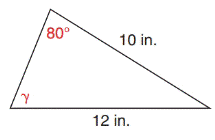Chapter 11.4, Problem 21EElementary Geometry For College St...

7th Edition
Alexander + 2 others
ISBN: 9781337614085

Solutions

Chapter
SectionElementary Geometry For College St...

7th Edition
Alexander + 2 others
ISBN: 9781337614085
Textbook Problem

In Exercises 17 to 22, use a form of the Law of Sines to find the measure of the indicated side or angle. Angle measures should be found to the nearest degree and lengths of sides to the nearest tenth of a unit.To determine

To find:

The angle of the given triangle by using the Law of Sines.

Explanation

Formula:

The Law of Sines,

sinαa=sinβb=sinγc

Where α, β, and γ is the acute angle of the triangle, and a, b, and c is the sides of the triangle.

Calculation:

Given,

From the triangle

b=12 in.,

c=10 in.,

β=80°

Find γ:

By the Law of Sines,

sinαa=sinβb=sinγc

Take,

sinαa=sinγc

Here, b=12 in., c=10 in., and β=80°

sin80°12=sinγ10

0

Still sussing out bartleby?

Check out a sample textbook solution.

See a sample solution

The Solution to Your Study Problems

Bartleby provides explanations to thousands of textbook problems written by our experts, many with advanced degrees!

Get Started

3. Graph the solution region for each of the following. (c)

Mathematical Applications for the Management, Life, and Social Sciences

Convert the expressions in Exercises 8596 radical form. x1/3y3/2

Finite Mathematics and Applied Calculus (MindTap Course List)

Use the guidelines of Section 4.5 to sketch the curve. y = 2 2x x3

Single Variable Calculus: Early Transcendentals, Volume I